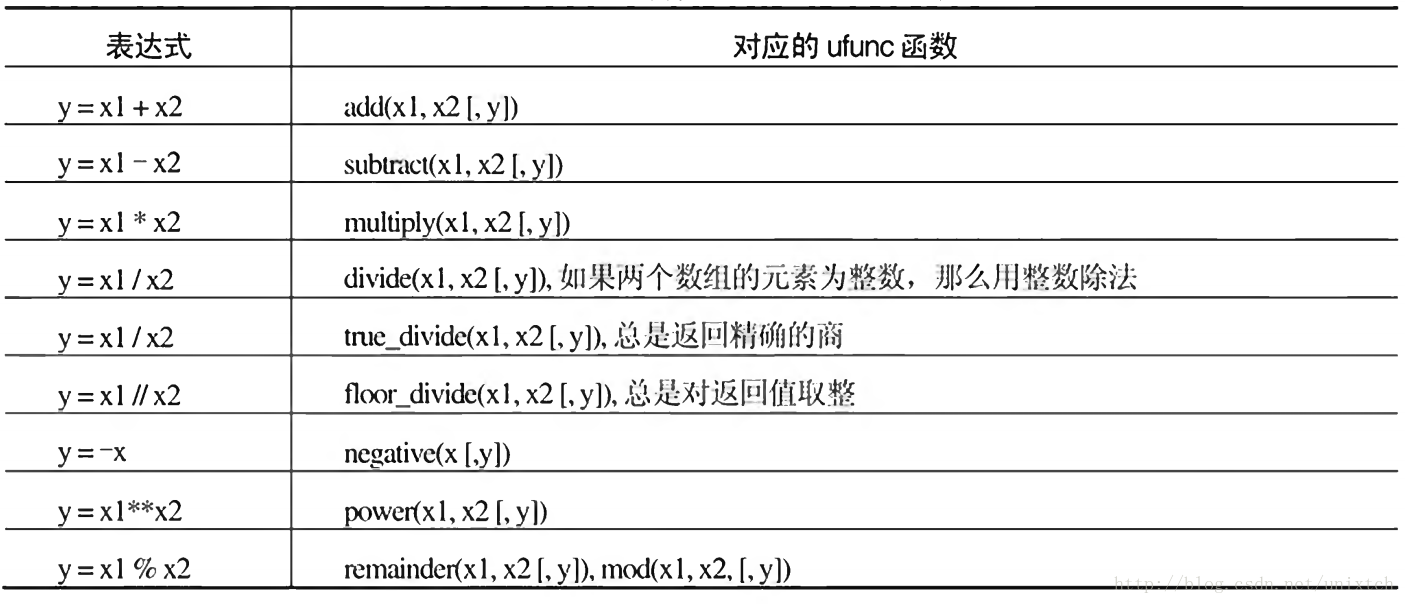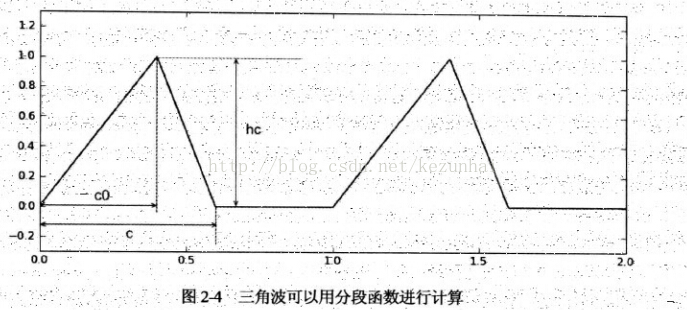# Shellblock

## 【Python】NumPy ufunc

ufunc是universal function的缩写，这些函数能够作用于narray对象的每一个元素上，而不是针对narray对象操作，numpy提供了大量的ufunc的函数。这些函数在对narray进行运算的速度比使用循环或者列表推导式要快很多，但请注意，在对单个数值进行运算时，python提供的运算要比numpy效率高。

### 广播机制

1. 让所有输入数组都向其中shape最长的数组看齐，shape中不足的部分都通过在前面加1(指1个维度)补齐；
2. 输出数组的shape是输入数组shape的各个轴上的最大值；
3. 如果各个输入数组的对应轴的长度相同或者其长度为1时，这样的数组之间能够用来计算，否则会出错；
4. 当输入数组的某个轴的长度为1时，沿着此轴运算时，使用此轴上的第一组值。

#### 一维ndarray广播

import numpy as np
arr1 = np.array([[0,0,0],[1,1,1],[2,2,2],[3,3,3]])
print(arr1)

arr2 = np.array([1,2,3])
print(arr2)

print(arr1 + arr2)


#### 二维ndarray广播

arr3 = np.arange(1,5).reshape(4,1)
print(arr3)

print(arr1+arr3)


A two dimensional array multiplied by a one dimensional array results in broadcasting if number of 1-d array elements matches the number of 2-d array columns.

### 常用ufunc运算

>>> a = np.arange(6.0).reshape(2,3)
>>> a.item(1,2)  #与a[1,2]类似
5.0
>>> type(a.item(1,2))
<class 'float'>
>>> type(a[1,2])
<class 'numpy.float64'>


#### 四则运算numpy提供的四则运算ufunc能够大大的提高计算效率，但如果运算式复杂，且参与运算的narray过大，会产生大量的中间结果，从而降低计算效率。例如：计算x=a*b+c时，实际上会按照如下方式计算：

t = a*b
x = t+c
del t


x = a*b
x = x+c


#### 比较和布尔运算

>>> np.array([1,2,3])==np.array([3,2,1])
array([False,  True, False], dtype=bool)
>>> np.array([1,2,3])<np.array([3,2,1])
array([ True, False, False], dtype=bool)


1. 编写对单个数值计算的目的函数；
2. 利用np.frompyfunc(func, nin, nout)将其转换为ufunc函数，其中func是上面编写的目的函数，nin是输入的参数个数，nout是返回值的个数。
## 基本形式
u_func = np.frompyfunc(func,nin,nout)
ret = u_func(narray_obj,param1,param2..)
1
2
3


## 基本形式
u_func = np.frompyfunc(func,otypes=[dtype1,dtype2..]
ret = u_func(narray_object,param1,param2..)


#### 自定义ufunc函数Python函数定义如下：

ef triangle_wave(x,c,c0,hc):
x = x - int(x)   #周期为1，取小数部分计算
if x>=c:
r = 0.0
elif x<c0:
r = x/c0*hc
else:
r = (c-x)/(c-c0)*hc
return r

##### 1.通过列表推导计算

x = np.linspace(0,2,1000)
y1 = np.array([triangle_wave(t,0.6,0.4,1.0) for t in x])

##### 2. fromnpyfunc( )函数计算

frompyfunc(func,nin,nout)


triangle_wave_ufunc = np.frompyfunc(triangle_wave,4,1)
y2 = triangle_wave_ufunc(x,0.6,0.4,1.0)


##### 3. vectorize( )函数计算

triangle_wave_vec = np.vectorize(triangle_wave, otypes[np.float])
y3 = triangle_wave_vec(x,0.6,0.4,1.0)


EOF